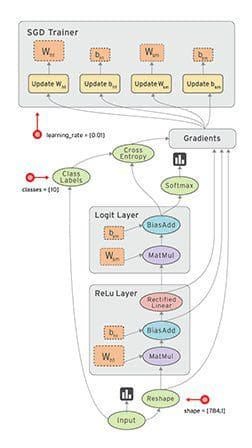# TensorFlow Disappoints – Google Deep Learning falls shallow

Google recently open-sourced its TensorFlow machine learning library, which aims to bring large-scale, distributed machine learning and deep learning to everyone. But does it deliver?commentsWhy was I disappointed with TensorFlow? It doesn't seem to fit any particular niche very well. Read on for the particulars. But first, let me get 2 things out of the way up front:

#1 - I am not a deep learning expert. I am currently pursuing a Master's thesis in machine learning, I read about, appreciate, and understand deep learning concepts and innovations, and implement nets in some of the leading frameworks, but I am not an expert.

#2 - I am an avowed supporter of Google, one might even say a "fan boy," in a way similar to how folks are Apple obsessed, or Microsoft manic (hey, there are probably a few).Moving on (for now)...

As announced last week, Google has open sourced TensorFlow, what it refers to as its second-generation system for large-scale machine learning implementations, and successor to DistBelief.

Built by the Google Brain team, TensorFlow represents computations as stateful dataflow graphs. TensorFlow is able to model computations on a wide variety of hardware, from consumer devices such as those powered by Android, to large-scale heterogeneous, multiple GPU systems. TensorFlow claims to be able to, without significant alteration of code, move execution of the computationally expensive tasks of a given graph from solely CPU to heterogeneous GPU-accelerated environments. Given these details, it goes without saying that TensorFlow aims to bring massive parallelism and high scalability to machine learning for all.

What follows is a general overview of TensorFlow, its architecture, and how it is utilized, after which I share my preliminary thoughts on the system.

TensorFlow Overview

At the heart of TensorFlow is the dataflow graph representing computations. Nodes represent operations (ops), and the edges represent tensors (multi-dimensional arrays, the backbone of TensorFlow). The entire dataflow graph is a complete description of computations, which occur within a session, and are executed on devices (CPUs or GPUs). Like much contemporary scientific computing and large-scale machine learning, TensorFlow favors its well-documented Python API, where tensors are represented internally as familiar numpy ndarray objects. TensorFlow relies on highly-optimized C++ for its computation, and also supports native APIs in C and C++.

Installation of TensorFlow is quick and painless, and can be accomplished easily with a `pip install` command. Once installed, getting a `Hello, TensorWorld!` program up and running is straightforward:
``````
import tensorflow as tf

# Say hello.
hello = tf.constant('Hello, TensorWorld!')
sess = tf.Session()
print sess.run(hello)
# --> Hello, TensorWorld!

# Some simple math.
a = tf.constant(10)
b = tf.constant(32)
print sess.run(a+b)
# --> 42
``````

Graphs are constructed from nodes (ops) that don't require input (source ops), which then pass their output to further ops which, in turn, perform computations on these output tensors, and so on. These subsequent ops are performed asynchronously and, optionally, in parallel. Notice the `run()` method calls above; they take, as arguments, the result variables for computations you are interested in performing, and a backward chain of required calls are made to achieve this end goal.

A further example illustrates randomly filling a pair of numpy ndarrays with floats, assigning these explicitly-created numpy ndarrays to tensorflow objects, and performing matrix multiplication on them. Note, in particular, the creation of a session and the use of the `run()` method. With no graph specified, TensorFlow uses the default instance.
``````
import tensorflow as tf
import numpy as np

# Pair of numpy arrays.
matrix1 = 10 * np.random.random_sample((3, 4))
matrix2 = 10 * np.random.random_sample((4, 6))

# Create a pair of constant ops, add the numpy
# array matrices.
tf_matrix1 = tf.constant(matrix1)
tf_matrix2 = tf.constant(matrix2)

# Create a matrix multiplication operation, pass
# the TensorFlow matrices as inputs.
tf_product = tf.matmul(tf_matrix1, tf_matrix2)

# Launch a session, use default graph.
sess = tf.Session()

# Invoking run() with tf_product variable will
# execute the ops necessary to satisfy the request,
# storing result in 'result.'
result = sess.run(tf_product)

# Now let's have a look at the result.
print result

# Close the Session when we're done.
sess.close()
``````

For more details on TensorFlow's implementation, see this whitepaper.

Experimenting with TensorFlow

In order to gain some appreciation for Google's newly open-sourced contribution to machine learning, I spent some time playing around with it. Specifically, I undertook as projects a few of the tutorials on the TensorFlow website. The tutorials are admittedly well-written, and though they explicitly, in more than one location, state that they are not generally suitable for learning machine learning, I'd argue that even a beginner would be able to read the documents and get something generalizable and useful from them.

The following are the specific tutorials I had a look at.

TensorFlow Mechanics 101

The goal of this tutorial is to show how to use TensorFlow to train and evaluate a simple feed-forward neural network for handwritten digit classification using the (classic) MNIST data set. The intended audience for this tutorial is experienced machine learning users interested in using TensorFlow.Learned embeddings using t-SNE.

Vector Representation of Words

In this tutorial we look at the word2vec model by Mikolov et al. This model is used for learning vector representations of words, called word embeddings.

Deep MNIST for Experts

TensorFlow is a powerful library for doing large-scale numerical computation. One of the tasks at which it excels is implementing and training deep neural networks. In this tutorial we will learn the basic building blocks of a TensorFlow model while constructing a deep convolutional MNIST classifier.

As stated above, all of the tutorials are well-written. They also performed without issue, and had results as advertised. However, I couldn't help feel a real sense of been here, done that. I understand the need for standardized introductions to topics, but beside being technically sound, it seemed like they were just going through the motions.